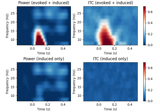# mne.minimum_norm.source_induced_power#

mne.minimum_norm.source_induced_power(epochs, inverse_operator, freqs, label=None, lambda2=0.1111111111111111, method='dSPM', nave=1, n_cycles=5, decim=1, use_fft=False, pick_ori=None, baseline=None, baseline_mode='logratio', pca=True, n_jobs=None, zero_mean=False, prepared=False, method_params=None, use_cps=True, verbose=None)[source]#

Compute induced power and phase lock.

Computation can optionally be restricted in a label.

Parameters:
epochsinstance of `Epochs`

The epochs.

inverse_operatorinstance of `InverseOperator`

The inverse operator.

freqs`array`

Array of frequencies of interest.

label`Label`

Restricts the source estimates to a given label.

lambda2`float`

The regularization parameter of the minimum norm.

method“MNE” | “dSPM” | “sLORETA” | “eLORETA”

Use minimum norm, dSPM (default), sLORETA, or eLORETA.

nave`int`

The number of averages used to scale the noise covariance matrix.

n_cycles

Number of cycles. Fixed number or one per frequency.

decim`int`

Temporal decimation factor.

use_fft`bool`

Do convolutions in time or frequency domain with FFT.

pick_ori`None` | “normal”

If “normal”, rather than pooling the orientations by taking the norm, only the radial component is kept. This is only implemented when working with loose orientations.

baseline`None` (default) or `tuple` of length 2

The time interval to apply baseline correction. If None do not apply it. If baseline is (a, b) the interval is between “a (s)” and “b (s)”. If a is None the beginning of the data is used and if b is None then b is set to the end of the interval. If baseline is equal to (None, None) all the time interval is used.

baseline_mode‘mean’ | ‘ratio’ | ‘logratio’ | ‘percent’ | ‘zscore’ | ‘zlogratio’

Perform baseline correction by

• subtracting the mean of baseline values (‘mean’)

• dividing by the mean of baseline values (‘ratio’)

• dividing by the mean of baseline values and taking the log (‘logratio’)

• subtracting the mean of baseline values followed by dividing by the mean of baseline values (‘percent’)

• subtracting the mean of baseline values and dividing by the standard deviation of baseline values (‘zscore’)

• dividing by the mean of baseline values, taking the log, and dividing by the standard deviation of log baseline values (‘zlogratio’)

pca`bool`

If True, the true dimension of data is estimated before running the time-frequency transforms. It reduces the computation times e.g. with a dataset that was maxfiltered (true dim is 64).

n_jobs

The number of jobs to run in parallel. If `-1`, it is set to the number of CPU cores. Requires the `joblib` package. `None` (default) is a marker for ‘unset’ that will be interpreted as `n_jobs=1` (sequential execution) unless the call is performed under a `joblib.parallel_backend()` context manager that sets another value for `n_jobs`.

zero_mean`bool`

Make sure the wavelets are zero mean.

prepared`bool`

If True, do not call `prepare_inverse_operator()`.

method_params

Additional options for eLORETA. See Notes of `apply_inverse()`.

use_cps`bool`

Whether to use cortical patch statistics to define normal orientations for surfaces (default True).

Only used when the inverse is free orientation (`loose=1.`), not in surface orientation, and `pick_ori='normal'`.

New in version 0.20.

verbose

Control verbosity of the logging output. If `None`, use the default verbosity level. See the logging documentation and `mne.verbose()` for details. Should only be passed as a keyword argument.

Returns:
power`array`

The induced power.

## Examples using `mne.minimum_norm.source_induced_power`#Compute power and phase lock in label of the source space

Compute power and phase lock in label of the source space